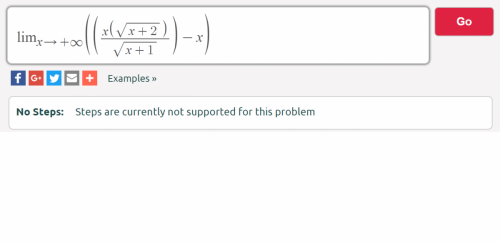Jump to content

# limit of ((x(sqrt(x+2)))/sqrt(x+1))-x is 1/2? Why?

## Recommended Posts

I need this limit ((x(sqrt(x+2)))/sqrt(x+1))-x to calculate the asymptote of this function: ((x(sqrt(x+2)))/sqrt(x+1)).

which, according to the class notes: y=x+1/2

with a = 1, b = 1/2

However, the online math calculators say that currently no steps are supported to show for this kind of problem.

I calculated this limit as x*sqrt(1)-x = 1, but apparently the correct answer is 1/2.

What is my mistake?

Thx in advance.

r##### Share on other sites

$\frac{x(\sqrt{x+2})}{\sqrt{x+1}}-x=\frac{x}{\sqrt{x+1}}(\sqrt{x+2}-\sqrt{x+1})= \frac{x}{\sqrt{x+1}(\sqrt{x+2}+\sqrt{x+1})}(x+2-(x+1))$

Limit = 1/2, since the denominator ->2x.

##### Share on other sites
• 1 month later...

You should use l'Hôpital's rule for a rigorous proof:
$\lim_{x\to\infty} {\frac{x\sqrt{x+2}}{\sqrt{x+1}}-x}=\lim_{x\to\infty} {\frac{x}{\sqrt{(x+1)(x+2)}+x+1}}$ (see the previous post).

Using l'Hôpital's rule, this equals $\lim_{x\to\infty} {\frac{1}{(\sqrt{(x+1)(x+2)})'+1}}=\lim_{x\to\infty} {\frac{1}{\frac{2x+3}{2\sqrt{(x+1)(x+2)}}+1}}$. Assuming the limit exists, this is equal to $\frac{1}{\lim_{x\to\infty} {\frac{2x+3}{2\sqrt{(x+1)(x+2)}}}+1}$.

You then evaluate $\lim_{x\to\infty} {\frac{2x+3}{2\sqrt{(x+1)(x+2)}}}$: Using l'Hôpital again, this is equal to $\lim_{x\to\infty} {\frac{2}{\frac{2(2x+3)}{2\sqrt{(x+1)(x+2)}}}}=\lim_{x\to\infty} {\frac{2\sqrt{(x+1)(x+2)}}{2x+3}}$. It follows that this limit is 1, and therefore $\lim_{x\to\infty} {\frac{x\sqrt{x+2}}{\sqrt{x+1}}-x}=\frac{1}{1+1}=\frac{1}{2}$.

Edited by renerpho
##### Share on other sites
• 3 months later...

Why use L'Hopital's rule? Mathematic's answer is much simpler.

##### Share on other sites

You can use wolframalpha to visualize the function, fyi:

https://www.wolframalpha.com/input/?i=((x(sqrt(x%2B2)))%2Fsqrt(x%2B1))-x

## Create an account or sign in to comment

You need to be a member in order to leave a comment

## Create an account

Sign up for a new account in our community. It's easy!

Register a new account

## Sign in

Already have an account? Sign in here.

Sign In Now
×

• #### Activity

• Leaderboard
×
• Create New...

## Important Information

We have placed cookies on your device to help make this website better. You can adjust your cookie settings, otherwise we'll assume you're okay to continue.